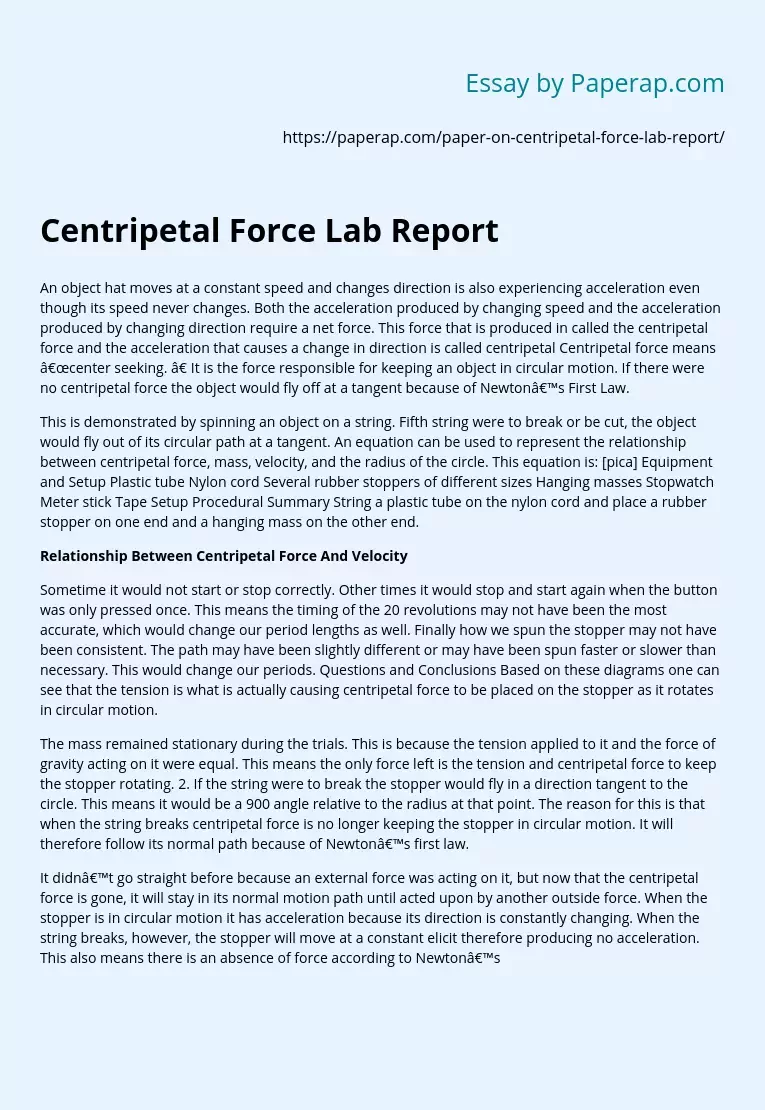# Centripetal Force Report

An object hat moves at a constant speed and changes direction is also experiencing acceleration even though its speed never changes. Both the acceleration produced by changing speed and the acceleration produced by changing direction require a net force. This force that is produced in called the centripetal force and the acceleration that causes a change in direction is called centripetal Centripetal force means “center seeking. ” It is the force responsible for keeping an object in circular motion. If there were no centripetal force the object would fly off at a tangent because of Newton’s First Law.

This is demonstrated by spinning an object on a string. Fifth string were to break or be cut, the object would fly out of its circular path at a tangent. An equation can be used to represent the relationship between centripetal force, mass, velocity, and the radius of the circle. This equation is: [pica] Equipment and Setup Plastic tube Nylon cord Several rubber stoppers of different sizes Hanging masses Stopwatch Meter stick Tape Setup Procedural Summary String a plastic tube on the nylon cord and place a rubber stopper on one end and a hanging mass on the other end.

## Relationship Between Centripetal Force And Velocity

Sometime it would not start or stop correctly. Other times it would stop and start again when the button was only pressed once. This means the timing of the 20 revolutions may not have been the most accurate, which would change our period lengths as well. Finally how we spun the stopper may not have been consistent.

Get quality help nowProf. FinchVerified

Proficient in: Force4.7 (346)

“ This writer never make an mistake for me always deliver long before due date. Am telling you man this writer is absolutely the best. ”+84 relevant experts are online

The path may have been slightly different or may have been spun faster or slower than necessary. This would change our periods. Questions and Conclusions Based on these diagrams one can see that the tension is what is actually causing centripetal force to be placed on the stopper as it rotates in circular motion.

The mass remained stationary during the trials. This is because the tension applied to it and the force of gravity acting on it were equal. This means the only force left is the tension and centripetal force to keep the stopper rotating. 2. If the string were to break the stopper would fly in a direction tangent to the circle. This means it would be a 900 angle relative to the radius at that point. The reason for this is that when the string breaks centripetal force is no longer keeping the stopper in circular motion. It will therefore follow its normal path because of Newton’s first law.

It didn’t go straight before because an external force was acting on it, but now that the centripetal force is gone, it will stay in its normal motion path until acted upon by another outside force. When the stopper is in circular motion it has acceleration because its direction is constantly changing. When the string breaks, however, the stopper will move at a constant elicit therefore producing no acceleration. This also means there is an absence of force according to Newton’s Second Law. 3. As centripetal force increased, the velocity increased. 4.

As the radius of the circle increased, the velocity increased. 5. As the mass of the moving stopper increased, the velocity decreased. 6. The centripetal force would need to decrease. This is because the radius is in the denominator and increasing the denomination with a constant numerator (mass and velocity) causes the quotient (centripetal force) to decrease. Conclusion This was a very successful lab overall. The graphs, the trend lines, and the slope of the trend lines really helped me to understand how changing the different factors in the centripetal force formula affects the results.

I was able to verify the relationships in a statistical and visual way. While not always the case, most of the time I was able to visually see how the velocity changed with each trial. This certainly helped me understand the relationship and trends between the variables. Our data was able to show a clear trend as well, so the error must have been minimal. It seems like the correlations of the lines of best fits re close to 1 or -1 as well, so this also shows OUr data had quite regular trends.